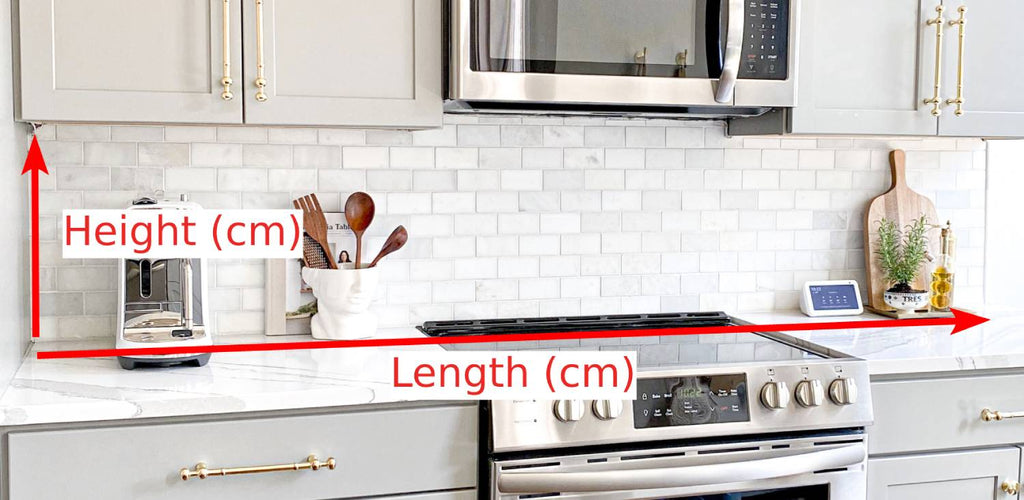# How to Measure Your Wall for Stick on TilesTo calculate the area in cm²:

Multiply the Height (cm) by the Length (cm)

Example: 60cm (Height) x 160cm (Length) = 9,600cm²

9,600 is the number you put in the box.

If you have multiple walls you want covered add the areas together.

Example: If your other area is 3,200cm², add it to 9,600cm² to get the new total = 12,800